### Vol 17, No 12 (2017) / Hu

Using Chebyshev polynomial interpolation to improve the computational efficiency of gravity models near an irregularly-shaped asteroid

# Using Chebyshev polynomial interpolation to improve the computational efficiency of gravity models near an irregularly-shaped asteroid

Hu Shou-Cun1, 2, Ji Jiang-Hui1,

CAS Key Laboratory of Planetary Sciences, Purple Mountain Observatory, Chinese Academy of Sciences, Nanjing 210008, China
University of Chinese Academy of Sciences, Beijing 100049, China

† Corresponding author. E-mail: jijh@pmo.ac.cn

Abstract: Abstract

In asteroid rendezvous missions, the dynamical environment near an asteroid’s surface should be made clear prior to launch of the mission. However, most asteroids have irregular shapes, which lower the efficiency of calculating their gravitational field by adopting the traditional polyhedral method. In this work, we propose a method to partition the space near an asteroid adaptively along three spherical coordinates and use Chebyshev polynomial interpolation to represent the gravitational acceleration in each cell. Moreover, we compare four different interpolation schemes to obtain the best precision with identical initial parameters. An error-adaptive octree division is combined to improve the interpolation precision near the surface. As an example, we take the typical irregularly-shaped near-Earth asteroid 4179 Toutatis to demonstrate the advantage of this method; as a result, we show that the efficiency can be increased by hundreds to thousands of times with our method. Our results indicate that this method can be applicable to other irregularly-shaped asteroids and can greatly improve the evaluation efficiency.

Keywords: minor planets, asteroids: individual (4179 Toutatis);methods: numerical;Chebyshev polynomials

## 1. Introduction

Asteroids are thought to be leftover planetesimals related to the precursor bodies which formed the planets in our solar system. Primitive asteroids may provide a record of the original composition of the solar nebula where the planets were born. The associated water and organic matter can provide us with important clues on the origin of life on Earth. In addition, near-Earth asteroids, whose orbits may cross Earthʼs orbit, may pose a potential risk to human beings on Earth (Michel et al. ).

Through ground- and space-based observations, and missions that flyby, rendezvous with and land on asteroids, as well as laboratory analysis of returned samples and all kinds of meteorites, we have made tremendous advances in knowledge about asteroids (Nesvorny et al. ). Among these techniques, space missions can directly acquire detailed information from the closest distances. Since the first close-up images of asteroid 951 Gaspra taken in 1991 by the Galileo spacecraft en route to Jupiter, 13 asteroids (including dwarf planets Ceres and Pluto) have been explored by spacecrafts. On 2012 December 13, the Chinese lunar probe Chang’e-2 flew by Toutatis at a surface distance of 0.77 km (Huang et al. ; Jiang et al. ; Zhao et al. ). Recently, OSIRIS-REx was launched by NASA on 2016 September 8 and is now on its way to asteroid 101955 Bennu (Lauretta & OSIRIS-Rex Team ). Moreover, the Hayabusa 2 mission, launched by JAXA in December 2014, will arrive at asteroid 162173 Ryugu in July 2018 (Müller et al. ). Both of these spacecrafts will bring sample dust from the asteroids back to Earth.

The gravitational field is essential to understand the dynamical environment of an asteroid, especially for the orbit design of spacecraft near asteroids (during the orbiting phase or landing phase). The images captured by spacecrafts have truly revealed the fact that most asteroids have irregular shapes, different from planets that approximate a spherical shape. The irregular shape of asteroids causes difficulty in calculating their gravitational field. Former investigations have shown that three major approaches of spherical/ellipsoidal harmonic expansion, polyhedral method and mascon approximation based on finite element representation have been developed to evaluate the gravity. Among them, the spherical harmonic method is based on series expansion (Kaula ; Lundberg & Schutz ; Hu et al. ), which may not converge inside the so-called Brillouin sphere (Brillouin ). Though ellipsoidal harmonic expansion has a larger convergence region (Romain & Jean-Pierre ; Garmier et al. ), the computation of ellipsoidal harmonics is not so straightforward and it does not fundamentally resolve the convergence problem. Recently, Takahashi & Scheeres () proposed using interior spherical harmonic expansion to extend the convergence region within the interior Brillouin sphere. However, this method is not suitable to be practically used due to its complexity. Assuming a constant density, the polyhedral method may be utilized to precisely evaluate the gravitational field (Werner & Scheeres ). Mascon approximation uses a collection of cubes or spheres to represent the true internal structure of asteroids (Park et al. ; Chanut et al. ; Zhao et al. ). However, both of them are computationally intensive, and the situation will get worse if the number of facets and vertexes or mascons increases. This problem is particularly acute for large-scale simulations (such as Monte Carlo analysis) or lower computational ability due to constraints on the size and weight of the onboard processor.

Several techniques have been proposed to minimize computation time in the polyhedral method, such as using simpler approximations to the more computationally intensive terms in the formula, or adopting a coarser shape model at the expense of accuracy (Cangahuala ; Weeks & Miller ). In this work, we introduce Chebyshev polynomial interpolation to accelerate the computational efficiency (Mason & Handscomb ), which has been widely used in numerical representation of planetary ephemerides for years, such as the DE-series of ephemerides developed at JPL and the INPOP ephemeris developed in France (Folkner et al. ; Fienga et al. ). Actually, it was initially put forward to speed up the calculation efficiency of Earthʼs gravity by Smith & Lyubomirsky (), in which Chebyshev expansions were applied only to the part of gravity force expressed by spherical harmonic terms of degree larger than four. However, the case for asteroids is quite different when considering the above-mentioned problem with convergence of harmonics. Herein we will refine this method to make it suitable to deal with irregularly-shaped asteroids by applying new schemes.

In Section 2, we will firstly introduce our method in detail, including the space partition method, comparison of the four interpolation schemes and error-adaptive octree division. In Section 3, we will show the computational efficiency and orbit integration precision with numerical simulations, by comparing the results with those of the polyhedral method. Finally, we present a brief conclusion.

## 2. Method

In mathematics, Chebyshev polynomials of the first kind are a sequence of orthogonal polynomials defined as solutions to the Chebyshev differential equation (Rivlin ). They may be calculated recursively as follows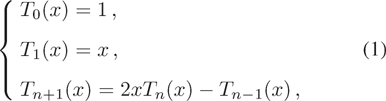where the range of x is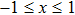. Chebyshev polynomials are stable during evaluation, and they provide a readily apparent estimate of previously neglected terms describing interpolation error. Besides high computational efficiency, the resulting interpolated polynomial also minimizes the problem of Runge’s phenomenon and provides an approximation that is close to the polynomial, which is the best approximation to a continuous function under the maximum norm (Hernández ).

As mentioned above, Chebyshev polynomials are widely used in numerical representation of planetary ephemerides (Newhall ). During the process, the range of time is segmented into contiguous intervals of fixed length and then the interpolation of rectangular coordinates is performed in each segment. Regarding gravitational acceleration near an asteroid, representing it as Chebyshev polynomials yields satisfactory results, except that we should consider three-dimensional Chebyshev polynomial interpolation in this situation. The basic formula is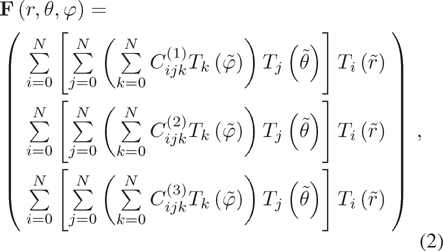where r, θ and φ are the three spherical coordinates in the body-fixed reference system, i.e. radial distance, longitude and latitude, respectively.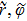and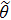are defined as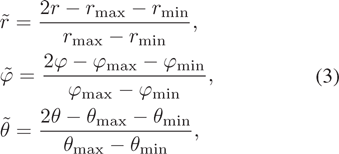where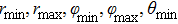and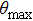are the minimal or maximal values of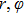and θ in the domain. T k are the Chebyshev polynomials defined in Equation (1).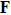is the gravitational acceleration vector and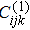,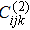and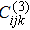are Chebyshev polynomial coefficients of each component with degree N (we have assumed the same degree in all three components), which may be solved by the least-squares method.

## 2.1. Division Scheme

In our method, the space near an asteroid is divided along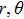and φ (we call it the spherical division scheme hereafter). Asteroid 4179 Toutatis is a typical irregularly-shaped asteroid, with dimensions x = 4.60 km, y = 2.29 km and z = 1.92 km (Hudson et al. ; Huang et al. ). Taking Toutatis as an example, the division is illustrated in Figure 1, where the range of each coordinate in each cell is represented as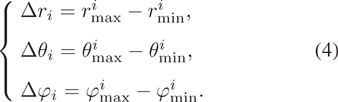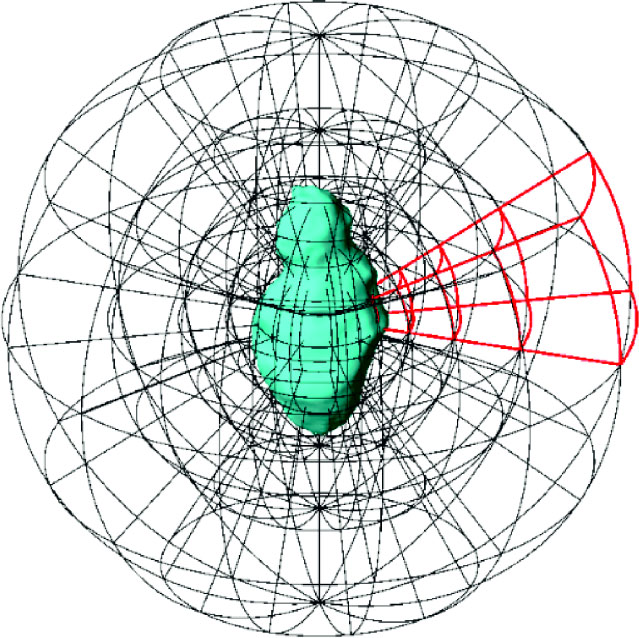Figure 1. An illustration of division of the neighborhood space along the spherical coordinate directions, taking asteroid 4179 Toutatis as an example. The red outlines show the division along some radial direction. The shape model is credited to Hudson et al. ().

In the illustration, the asteroid is divided uniformly along the longitude and latitude directions (let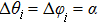). However, in the radial direction the range is picked so that it is nearly proportional to radial distance, i.e.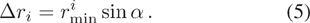The trick above is based on the fact that the variation of gravitational acceleration is gentler at further distance, so we can use larger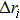for larger r, which reduces the amount of storage required for coefficients. The error from interpolation along the radial direction will be shown hereinafter. In this scheme, we can use α and N to adjust the precision (both smaller α and larger N may reduce the error, but demands larger storage), and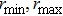to constrain the domain we are interested in. Of course,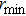is usually chosen as the minimal radial distance at the surface.

In programming, we only need to load the coefficients once, and then the computation time of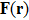almost only depends on N (as we can see in Eq. (2), the calculation is not related to α). The whole procedure includes generation of coefficients and calculation of gravity. The polyhedral method is used during the process of generating coefficients.

## 2.2. Comparison of Four Interpolation Schemes

As is well known, gravitational acceleration can be divided into central and non-spherical parts. Thus Equation (2) is modified as (Kaula )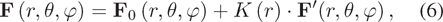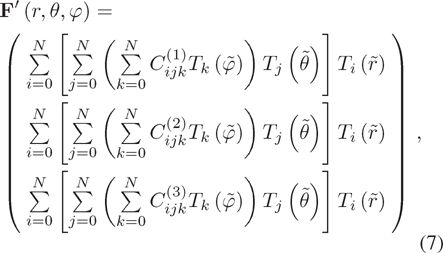where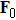is the part that can be calculated analytically and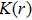is a scalar coefficient related to r. Let us consider the three different schemes (denoted as I1, I2 and I3)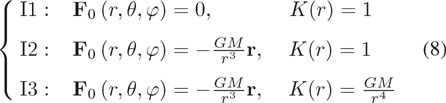where I1 is the same as Equation (2), but I2 and I3 only fit the non-spherical part. I3 also considers characteristics that generally include the largest zonal and tesseral harmonics describing gravitational acceleration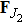and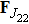, which are inversely proportional to the fourth power of r (Kaula )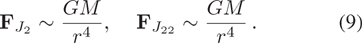In addition, we may consider another interpolation scheme. In Equations (6), (7) and (8), the gravitational acceleration is represented with rectangular components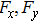and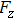by default. However, we can also try to transform them to three components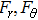and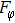along spherical coordinates, i.e.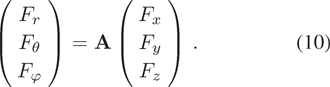Here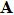is the transformation matrix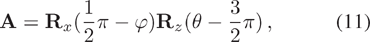in which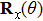and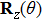are defined as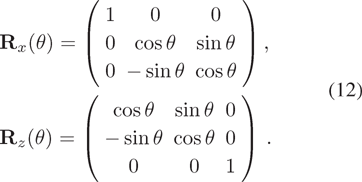Moreover, we use the same value of scheme I3 for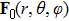and. This is denoted as scheme I4.

Now, let us compare the interpolation results for Toutatis. Set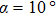and N = 2,3,4,5. The relative error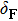is defined as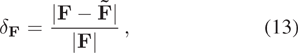whereis the gravitational acceleration calculated with the polyhedral method (see eq. (15) in Werner & Scheeres ) and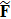is the value by interpolation. The comparisons offor the four interpolation schemes are shown in Figure 2, wherevaries with r.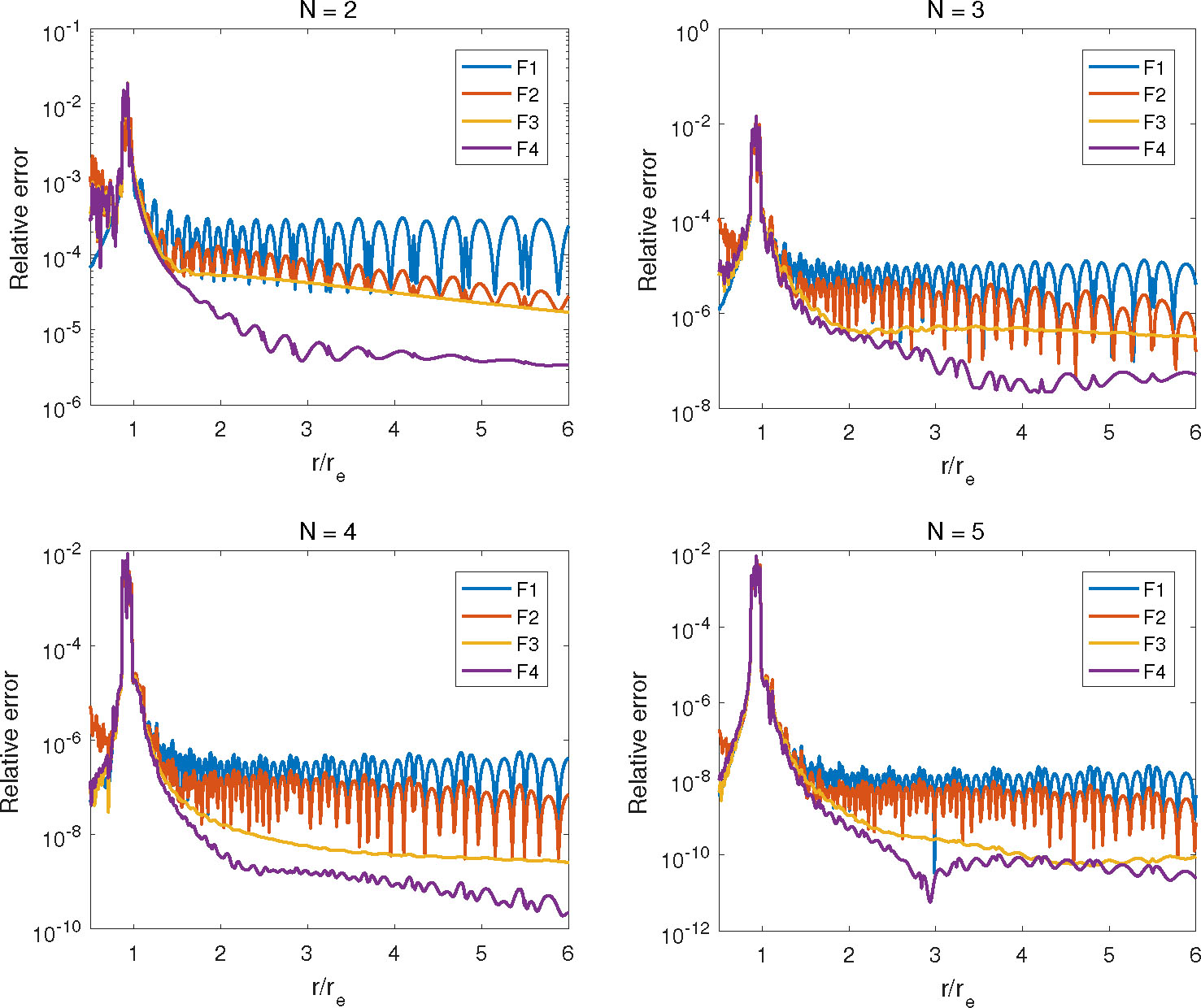Figure 2. The relative error vs. r for four interpolation schemes I1, I2, I3 and I4 in direction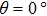and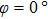. The degrees of Chebyshev polynomials are 2, 3, 4 and 5, as labeled at the top of each panel. r e is radial distance on the surface.

In the results, relative errors reach maximum near the surface, which is because of the abrupt gravity change in this area. Anyway, we can see that I1 has the best result inside the asteroid. But outside, I3 is better than I1 and I2, while I4, plus a transformation on I3, has a better result than I3, especially for lower degree N. Because we only care about gravity outside the asteroid for most situations, here we recommend using the I4 scheme in our method.

## 2.3. Comparison between Spherical and Rectangular Division Schemes

As Figure 2 shows, for all four schemes, the relative errors generally do not increase with r when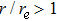, by which we show that the linear increase in radial range of each cell in Equation (5) is a reasonable choice for our spherical division scheme. As mentioned above, this will reduce the required amount of storage for large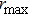. Actually, we may estimate the amount of storage for Chebyshev coefficients for a specific α and N. As illustrated in Figure 3, the speed of storage increment withis decreasing as r increases. That means we do not need to worry about the storage of coefficients too much when we need to consider a large. Besides, as we can see in Figure 2, the error is satisfactory at large r. So, we may not need to replace our method by switching to use spherical harmonic expansion to calculate the gravity at large r.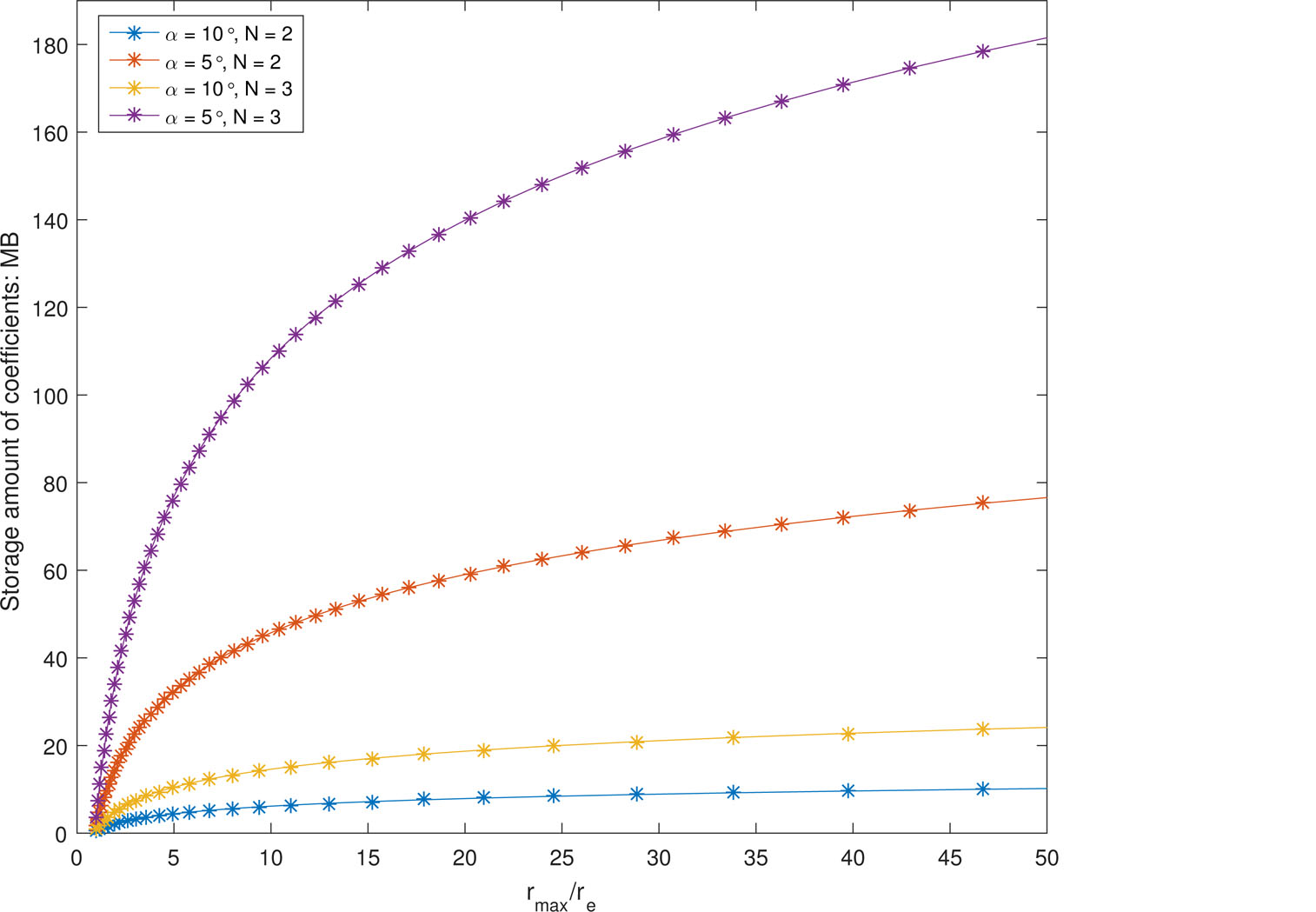Figure 3. The amount of storage required for Chebyshev coefficients vs.(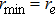) (double-precision binary floating-point is assumed for the storage of coefficients).

Despite the advantage above, we are still interested in the comparison between spherical division and rectangular division. For the latter, the division is along the directions of three rectangular coordinates x, y and z, in which the cells are actually cubes (assuming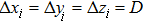for all the cells) in a rectangular coordinate system.

Consider three different cells near Toutatis, which are divided in a spherical way with, as illustrated in the top panel of Figure 4, in which A, B and C are the centers of each cell and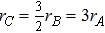. Only cell A crosses the interior and exterior parts of Toutatis. The red outline is the profile of the three cells projected in plane y = 0. We can also have a rectangular division at A, B and C, with the length of each cube equaling the average scale of cells A, B and C, respectively. They are illustrated as the black outline in the top panel.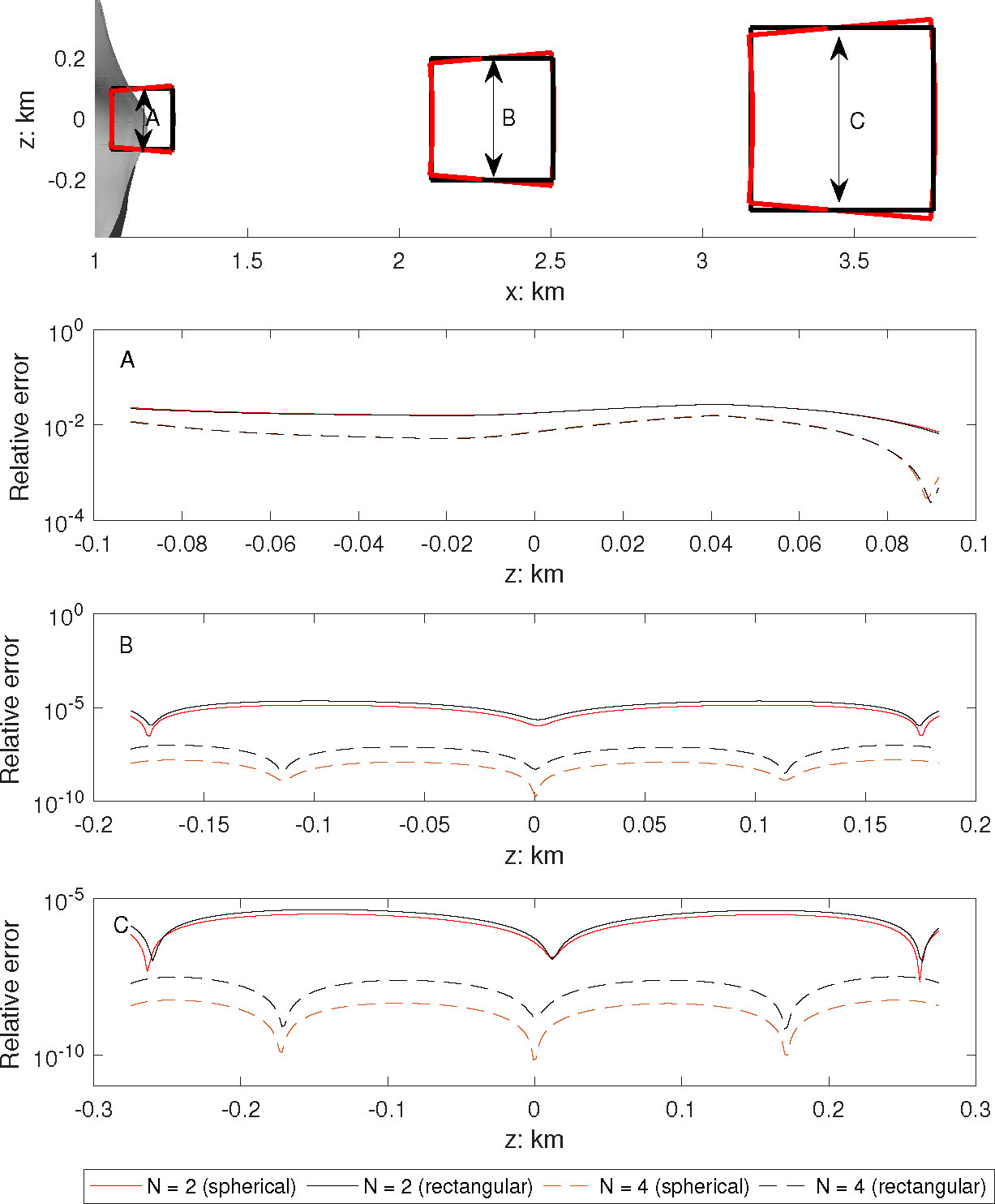Figure 4. The comparison of relative errors for spherical and rectangular division schemes in areas A, B and C. The I4 interpolation scheme is adopted in the calculation.

The relative errors along the z-axis (with position along the double arrows) crossing each center of A, B and C are displayed in Figure 4, where N = 2, 4 and both spherical and rectangular division results are exhibited. We can see that the two division schemes show almost no difference in area A, but spherical division prevails in areas B and C (for area B, the mean relative error ratios of spherical to rectangular division are 0.6 and 0.16 for N = 2 and N = 4, respectively, and for area C, the values are 0.72 and 0.18). This experiment concludes that the spherical division scheme has an advantage over rectangular division far from the surface of the asteroid; this is another reason we recommend using spherical division.

## 2.4. Combined with Octree Division

We have done numerical experiments to demonstrate that I4 interpolation and the spherical division scheme are good choices when we use Chebyshev polynomials to approximate gravity near an irregularly-shaped asteroid. Setting N = 2 and α = 10°, the profiles of relative errors of Toutatis in the planes y = 0 and x = 0 are shown in Figure 5. We can see that the relative error may increase to ∼0.1 near the surface and decreases to less than 0.001 for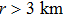, which is consistent with the results given in Figure 2. To improve the precision, we may reduce the size of each cell by decreasing α or increasing the degree N. The experiments are performed for areas A, B and C in Figure 4. We fix N = 2 and halve α from 20° to 1.25°. Then, we fix α = 10° and increase N from 2 to 6. Their results of maximal relative errors are shown in Tables 1 and 2, respectively.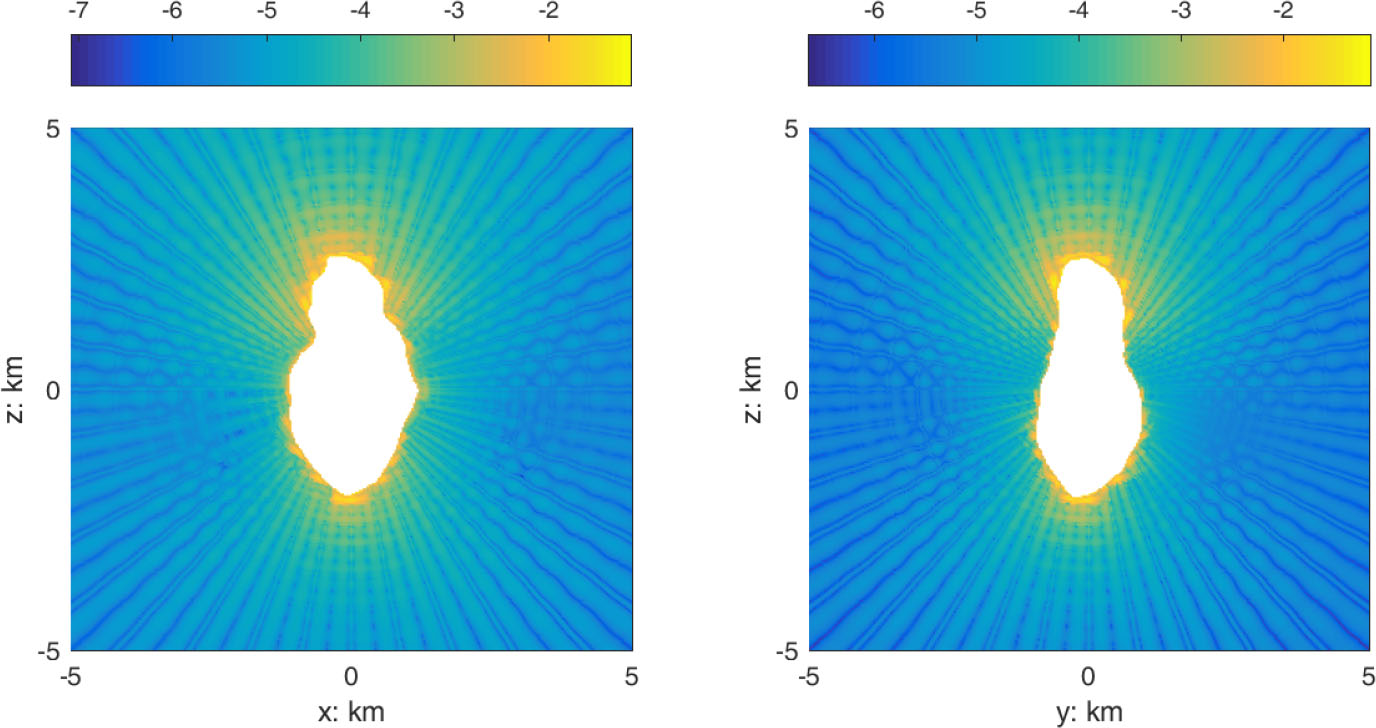Figure 5. The profiles of relative errors at y = 0 (left) and x = 0 (right) near asteroid Toutatis, where N = 2 and. We have applied the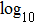operation to the relative error. The interior gravity is not calculated.
α = 20° α = 10° α = 5° α = 2.5° α = 1.25°
A 1.09E–01 5.20E–02 2.23E–02 1.47E–02 8.82E–03
B 6.36E–04 5.55E–05 5.84E–06 6.72E–07 8.06E–08
C 1.89E–04 1.92E–05 2.18E–06 2.61E–07 3.21E–08
Table 1.

The maximal relative errors of cells A, B and C. N = 2 and α varies from 20° to 1.25°.

N = 2 N = 3 N = 4 N = 5 N = 6
A 1.13E–02 1.11E–02 6.36E–03 6.12E–03 3.91E–03
B 2.92E–06 7.63E–08 6.21E–10 2.70E–11 3.58E–13
C 1.09E–06 2.55E–08 1.51E–10 2.42E–12 4.99E–14
Table 2.

The maximal relative errors of cells A, B and C. α = 10° and N increases from 2 to 6.

The results show that for each halving of α, the maximal errors of B and C are reduced by 810 times, while the value is up to 50 times for each increase of N from 2 to 6 on average. But the situation is totally different for A, where the errors are only reduced by less than 0.5 times for each halving of α, and the situation is even worse for an increase of N. As an illustration, the variations of F r with z in plane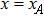for different y are shown in Figure 6, where the dashed lines mean the locations are inside the asteroid. The variations of F r with z are continuous curves, but obvious jumps occur at the adjacent area. So, it is a natural result for area A when we use a smooth curve (Chebyshev polynomials) to fit the not-so-smooth gravity. If we need to refine the precision near the surface, a natural choice is to only reduce the cell size in these areas (we do not choose to increase the degree N because this will further burden computation time, as we can see in Table 3), but not reduce α globally, because the latter one will unnecessarily reduce the cell size far away from the surface and greatly increase the needed storage (a rise of 8 times in storage for each halving of α).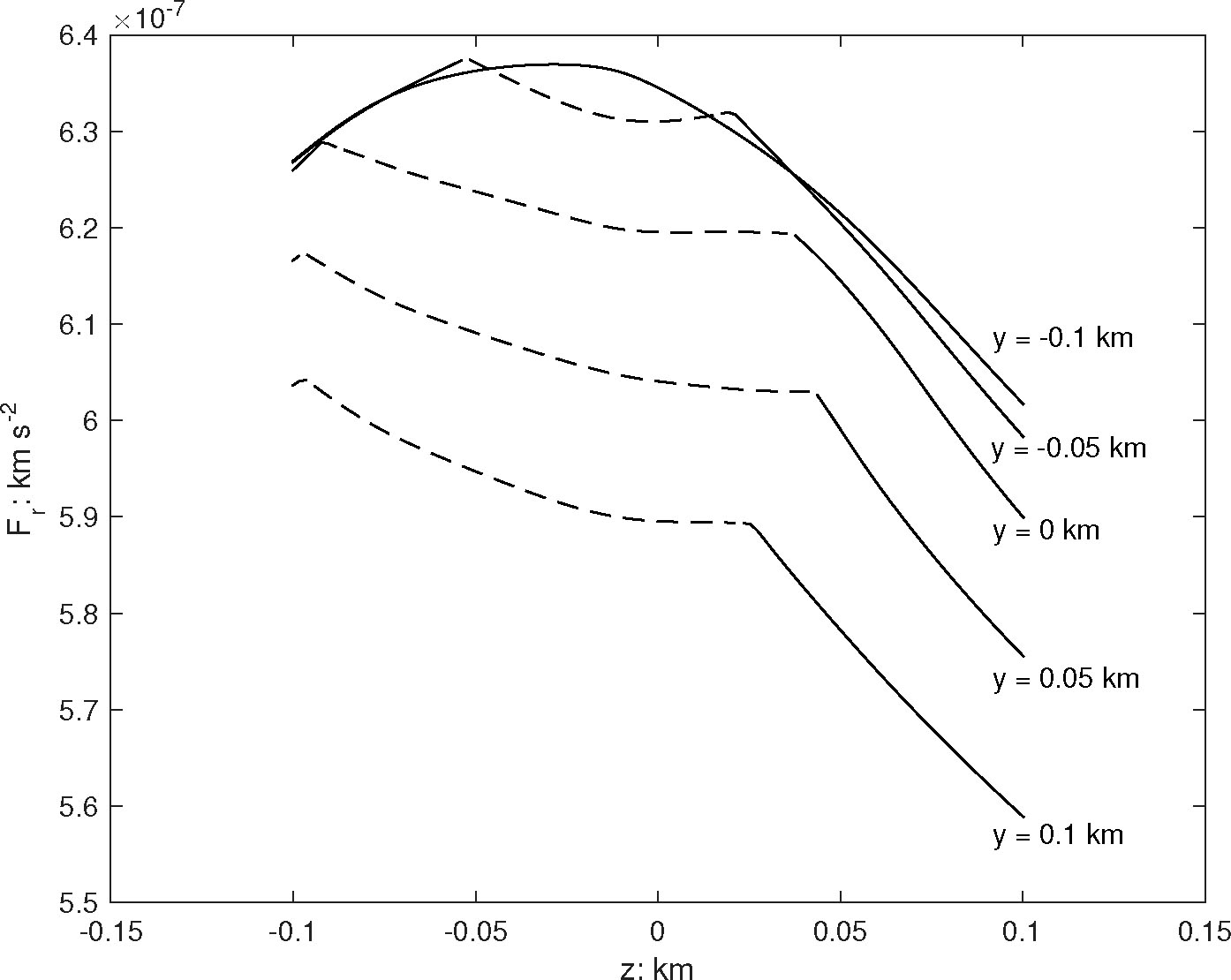Figure 6. F r vs. z in planefor different y. The solid and dashed lines represent the points being outside or inside the asteroid Toutatis, respectively.
Method Polyhedral N = 2 N = 3 N = 4 N = 5 N = 6
Time consumption 1.0 7.6E–4 1.2E–3 1.7E–3 2.4E–3 3.2E–3
Table 3.

The Ratio of Computation Time for Our Method to that of the Polyhedral Method (α = 10° and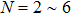).

With the above results in mind, the spherical division scheme is refined by combining with adaptive octree division (Frisken & Perry ), in which an error tolerance (denoted as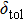) is set in addition to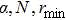and. The maximal relative error (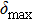) of each cell is evaluated during the division. If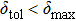, then the cell will be divided by half and this process is repeated recursively. Finally the cells may have different sizes and they are managed by an octree data structure. The whole procedure of coefficient generation and gravity calculation in our method is illustrated in Figure 7.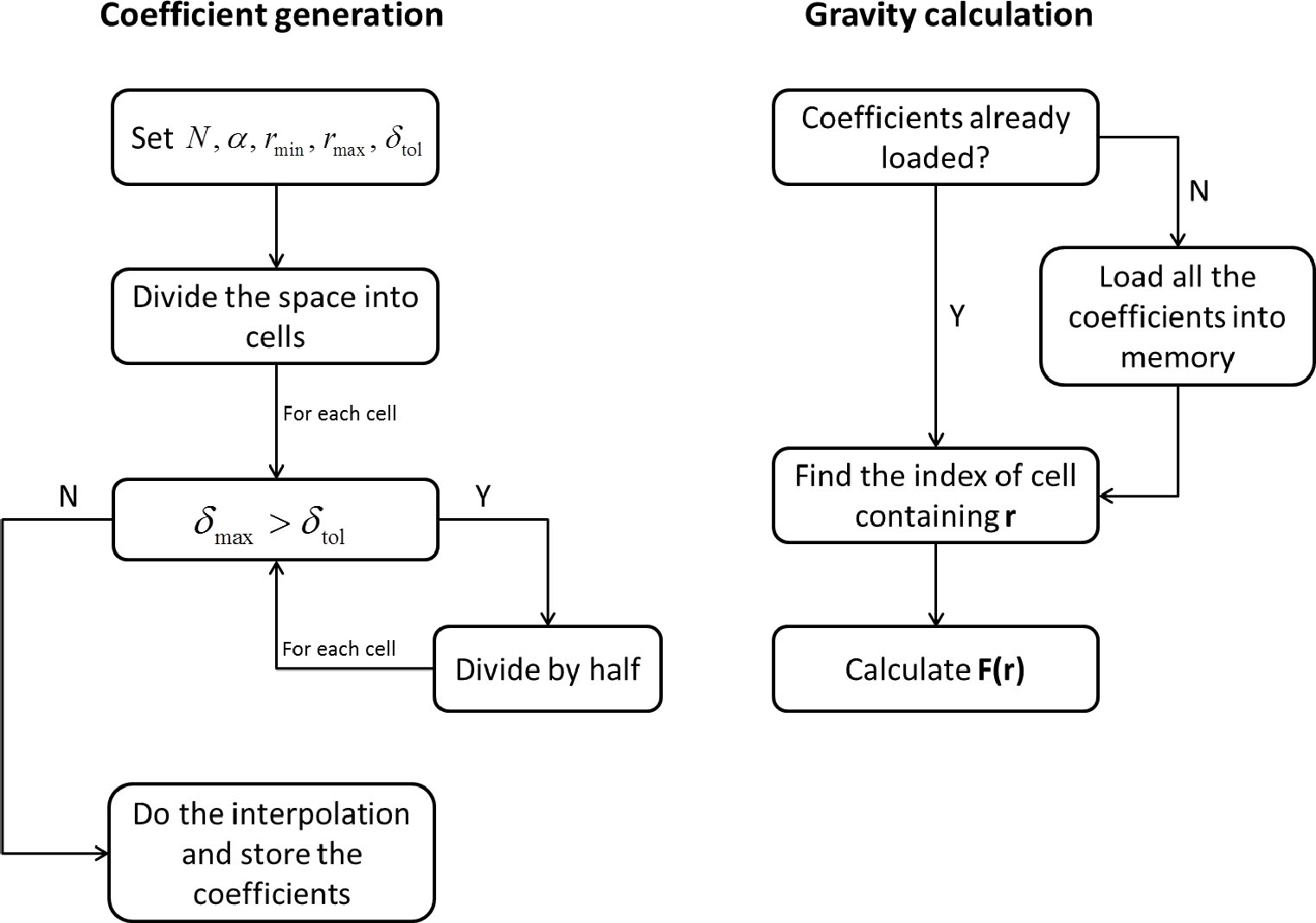Figure 7. The basic programming procedure for coefficient generation and gravity calculation. The polyhedral method is used in coefficient generation.

As an experiment, we set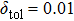, and then Figure 5 was refined as displayed in Figure 8, where the maximal relative errors near the surface have been reduced to less than 0.01.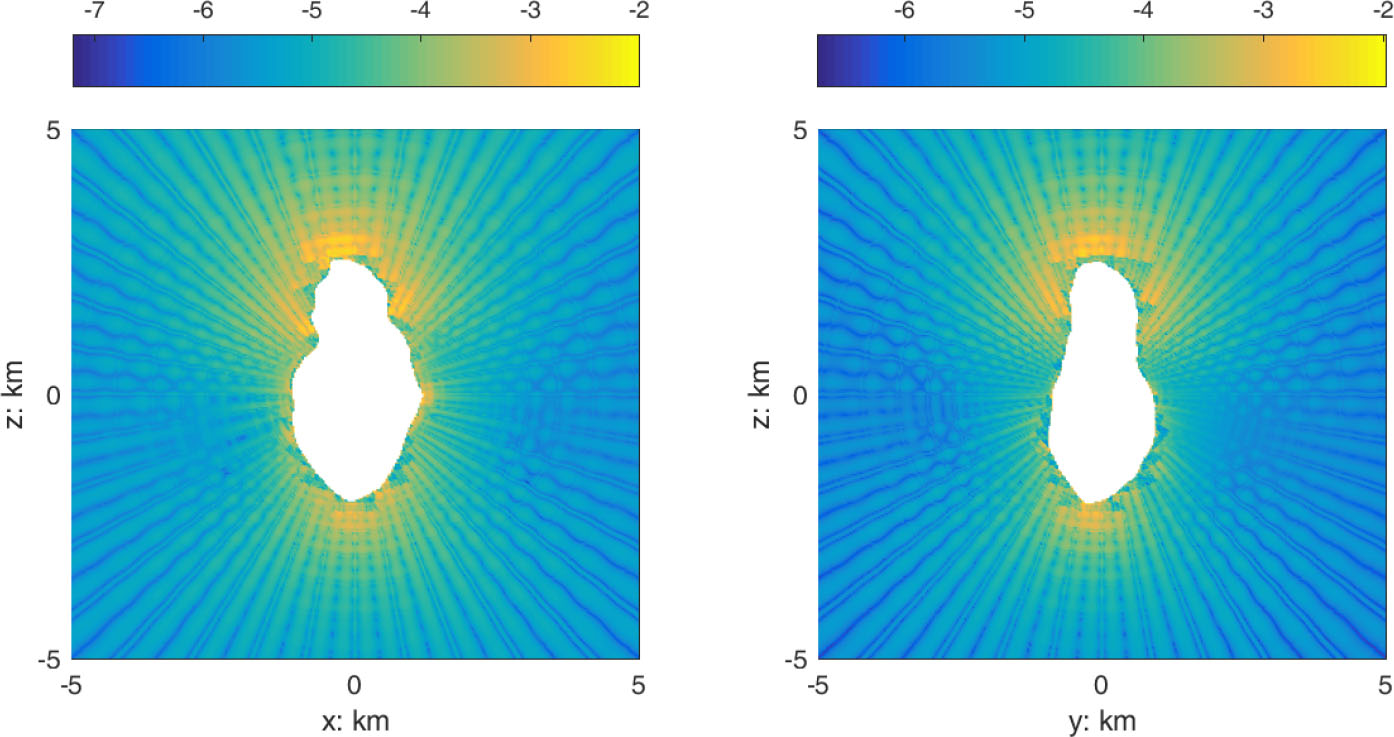Figure 8. The profiles of relative errors at y = 0 (left) and x = 0 (right) near asteroid Toutatis, where N = 2 and α = 10°. The interior gravity is not calculated.

## 3. Computational Efficiency and Precision of Orbit Integration

Based on the procedures illustrated in Figure 7, we are able to generate the coefficients for asteroid Toutatis. Two important aspects of this method we are concerned with are computational efficiency and error of orbit propagation.

## 3.1. Comparison of Computational Efficiency with the Polyhedral Method

In our computation, the number of facets and vertexes of Toutatis’ shape model are 6400 and 12 796 respectively (Hudson et al. ). After loading all the coefficients into memory, the computational efficiency almost only depends on degree N. Compared with the polyhedral method, the relative elapsed time foris listed in Table 3.

The results tell us that the computational efficiency of our method is hundreds to thousands of times higher than the polyhedral method, and this advantage is more prominent for shape models with larger numbers of facets and vertexes. As N increases, the computation time also increases slightly. So, in the sense of efficiency, for the same precision, we recommend using small N and small α, but not large N and large α.

## 3.2. Error Comparison of Orbit Propagation with the Polyhedral Method

In most situations, the computation of gravitational acceleration is used in orbit integration. It is interesting to compare the orbit integration error between our method and the polyhedral method.

Computing gravity near the surface of an asteroid is essential for proximation operations (including hovering, landing and touch-and-go maneuvers) of spacecrafts. To show the application of our method in this circumstance, we have integrated 10 000 orbits of ejecta particles randomly launched from the surface of Toutatis with a launch angle of 45° and velocities of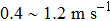(the average escape velocity of Toutatis on the surface is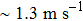) by using our method and the polyhedral method to calculate the gravitational acceleration. Only particles that re-impact the surface are recorded. About 5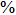of particles have orbit times larger than 1 day, whose eccentricities are so large that their orbital errors are very sensitive to the gravity. So, these particles are rejected because they are not suitable for comparison. For the remaining 95% of particles, a histogram showing the distribution of position errors is displayed in Figure 9, where N = 2, and α = 10° or 5°. The dashed lines meanis not set while solid lines represent cases having. We can see that the precision has an obvious enhancement after combining with the adaptive octree division, in which about 94.9% and 97.6% of particles have errors less than 0.01 km for α = 10° and α = 5°, respectively.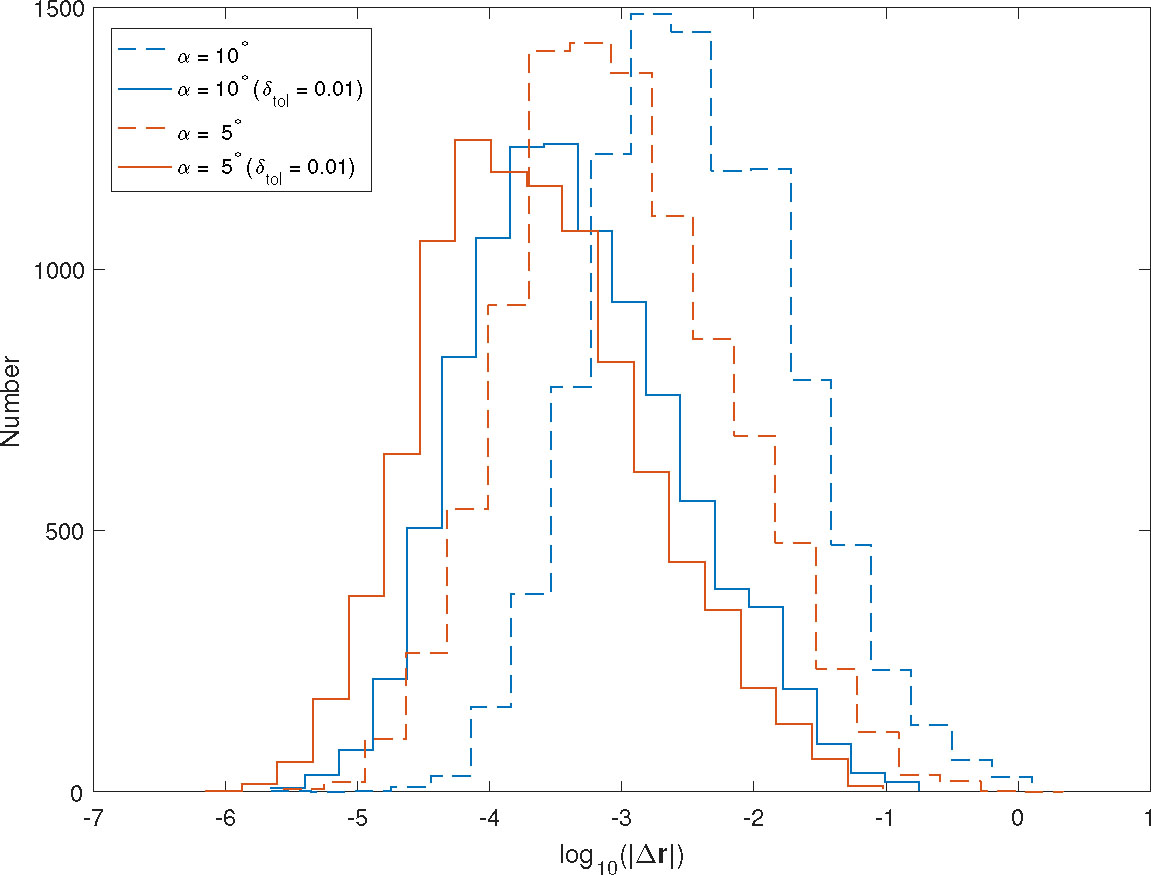Figure 9. The distribution of orbit errors for ejecta particles. N = 2 and α = 10° or 5°. The dashed lines represent cases in which the value ofis not set, but solid lines refer to cases in which.

Another situation we are concerned with is stable motion around the asteroid. In most cases, these orbits are high enough that we can safely use harmonic expansions to calculate the gravity. But this will bring additional trouble related to retrieval of harmonic coefficients. Nevertheless, here we would like to perform experiments only using our method. 10 000 particles are placed in circular orbits with r = 4 km (about twice the asteroid radius) with randomly picked inclinations and mean anomalies. The propagation is performed for 10 cycles (about 5.6 d). Using the same parameters as in Figure 9, the results are shown in Figure 10. This time, the dashed lines and solid lines coincide because the adaptive octree division ofdoes not influence the domains through which these orbits pass. About 99.9% and 79.1% of orbits have errors less than 0.01 km for α = 5° and α = 10°, respectively.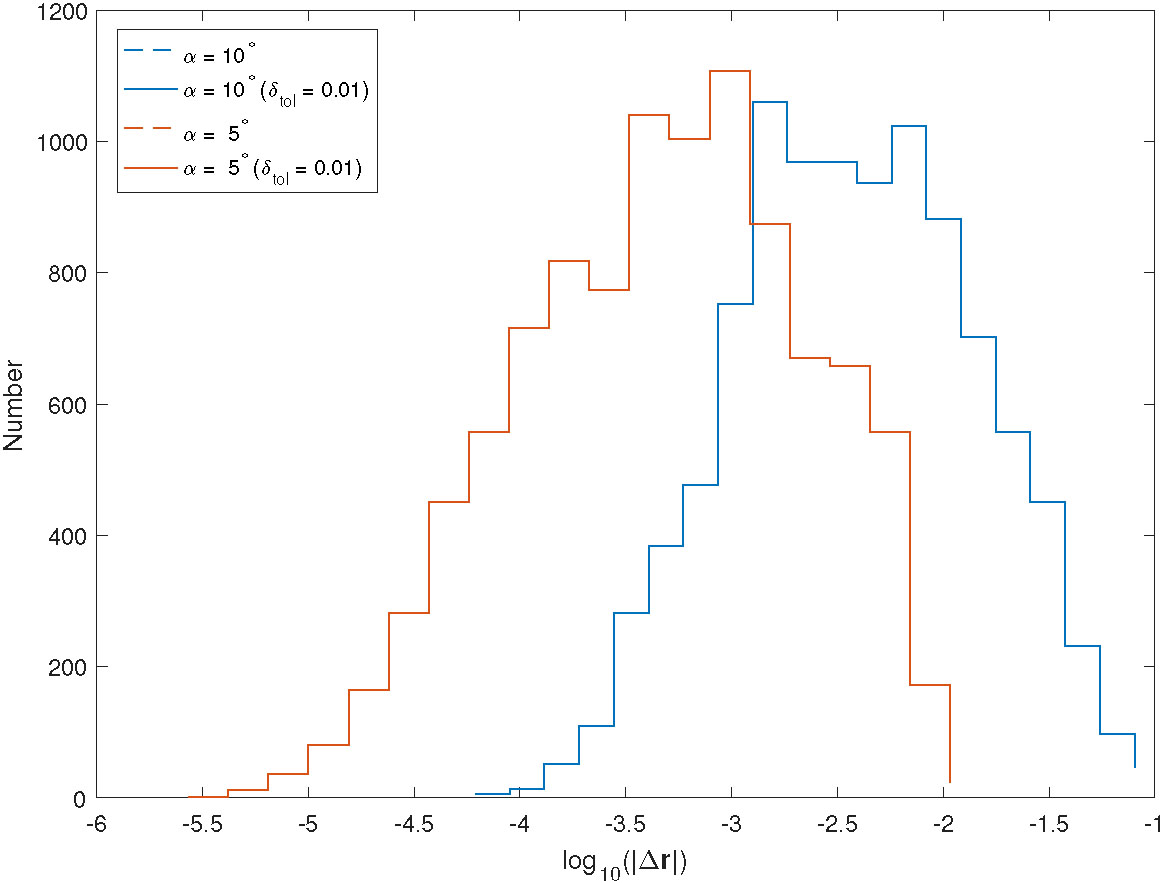Figure 10. The distribution of orbital errors for circular orbits with r = 4 km. The other parameters are the same as in Fig. 9.

These two experiments indicate that decreasing the cell size may definitely reduce the orbital error. For orbits near the surface, using adaptive octree division is necessary and we can see it works well for orbit integration. The orbital errors for N = 2, α = 5° andare acceptable in some situations, such as Monte Carlo simulation, or preliminary orbit design. But if you still need higher precision, reducingmay be a good choice. However, please keep in mind that the rate of error reduction near the surface is very small as α decreases, which means you probably need a very high cost for storage of coefficients, which is a trade off for a little enhancement in precision.

Finally, for reference about storage, if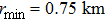,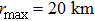and ignoring the cells totally inside Toutatis, the double-precision binary storage required for coefficients is 54.7 MB and 7.4 MB for α = 5° and 10° withnot set, respectively, while the values are 107.4 MB and 61.6 MB with, respectively.

## 4. Conclusions

In this article, we propose using Chebyshev polynomial interpolation to increase the computational efficiency of gravitational acceleration near an irregularly-shaped asteroid, in which the gravity of the neighborhood domain of the asteroid is precomputed by a computationally expensive polyhedral method and the interpolation coefficients are stored. Spherical division and rectangular division schemes, and four interpolation schemes on different components of gravitational acceleration, are both compared, and we recommend adopting spherical division and the I4 interpolation scheme according to numerical experiments performed on asteroid 4179 Toutatis. The spherical division we propose along the radial direction is not uniform, where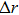of each cell is nearly proportional to the radial distance. It allows us to use our method to calculate the gravity globally for some orbits not too far away from the surface at the cost of not too much additional storage increment. The I4 interpolation scheme suggests representing gravitational acceleration along the three spherical coordinate directions, and we only need to apply the interpolation on the non-spherical part with an extra consideration about characteristic variation along the radial direction.

After that we show the computational efficiency may be enhanced by hundreds to thousands of times for the typical asteroid Toutatis and the enhancement in speed mainly depends on the degree of the polynomials. Orbit propagation experiments are performed for 10 000 ejecta orbits and stable midrange orbits. The results tell us that we can obtain a generally acceptable orbit precision by simply setting the parameters N = 2, α = 5° and, and the amount of storage required for coefficients is also acceptable.

However, we also notice that there is an obvious balance between precision and amount of storage required for coefficients. Special concern is noted about the slow error convergence near the asteroid surface; this is a drawback when using Chebyshev polynomial interpolation, for which an abrupt gravity change near the surface greatly increases the interpolation error. Subsequent improved research should focus on this issue.

 Brillouin M. 1933 Equations aux Dériveées partielles du 2e ordre. Domaines à connexion multiple Fonctions sphériques non antipodes Annales De LInstitut H. Poincaré 4 173 Cangahuala L. A. 2005 Augmentations to the polyhedral gravity model to facilitate small body navigations American Astronautical Society Paper 05-146 Chanut T. G. G. Aljbaae S. Carruba V. 2015 MNRAS 450 3742 Fienga A. Manche H. Laskar J. Gastineau M. 2008 A&A 477 315 Folkner W. M. Williams J. G. Boggs D. H. Park R. S. Kuchynka P. 2014 Interplanet. Netw. Prog. Rep 196 1 Frisken S. F. Perry R. N. 2002 Journal of Graphics Tools 7 1 Garmier R. Barriot J.-P. Konopliv A. S. Yeomans D. K. 2002 Geophysical Research Letters 29 Hernández M. 2001 Computers & Mathematics with Applications 41 433 Hu S.-C. Ji J.-H. Zhao Y.-H. 2015 RAA (Research in Astronomy and Astrophysics) 15 896 Huang J. Ji J. Ye P. et al. 2013 Scientific Reports 3 3411 Hudson R. S. Ostro S. J. Scheeres D. J. 2003 Icarus 161 346 Jiang Y. Ji J. Huang J. et al. 2015 Scientific Reports 5 16029 Kaula W. M. 1966 Theory of Satellite Geodesy Applications of Satellites to Geodesy Waltham, Mass. Blaisdell Lauretta D. S. OSIRIS-Rex Team 2012 An overview of the Osiris-rex Asteroid Sample Return Mission Lunar and Planetary Science Conference 43 2491 Lundberg J. Schutz B. 1988 Journal of Guidance, Control, and Dynamics 11 31 Mason J. C. Handscomb D. C. 2002 Chebyshev polynomials CRC Press Michel P. DeMeo F. E. Bottke W. F. 2015 Asteroids: Recent Advances and New Perspectives Michel P. DeMeo F. E. Bottke W. F. Asteroids IV Tucson University of Arizona Press 3 Müller T. G. Ďurech J. Ishiguro M. et al. 2017 A&A 599 A103 Nesvorny D. Brož M. Carruba V. 2015 Asteroid IV Michel P. DeMeo F. E. Bottke W. Tucson University of Arizona Press Newhall X. 1988 Celestial Mechanics 45 305 Park R. S. Werner R. A. Bhaskaran S. 2010 Journal of Guidance, Control, and Dynamics 33 212 Rivlin T. 1990 Chebyshev Polynomials: from Approximation Theory to Algebra and Number Theory Pure Appl. Math. NY Romain G. Jean-Pierre B. 2001 Celestial Mechanics and Dynamical Astronomy 79 235 Smith R. Lyubomirsky A. 1981 Techniques for Increasing the Efficiency of Earth Gravity Calculations for Precision Orbit Determination, in In NASA Goddard Space Flight Center Sixth Ann. Flight Mech./Estimation Theory Symp. 28 (SEE N82-10064 01-12), 1 Takahashi Y. Scheeres D. 2014 Celestial Mechanics and Dynamical Astronomy 119 169 Weeks C. Miller J. K. 2004 Advances in the Astronautical Sciences 112 553 Werner R. A. Scheeres D. J. 1996 CelestialMechanics and Dynamical Astronomy 65 313 Zhao Y.-H. Hu S.-C. Wang S. Ji J.-H. 2016 Chinese Astronomy and Astrophysics 40 45 Zhao Y. Ji J. Huang J. et al. 2015 MNRAS 450 3620
Cite this article: Hu Shou-Cun, Ji Jiang-Hui. Using Chebyshev polynomial interpolation to improve the computational efficiency of gravity models near an irregularly-shaped asteroid. Res. Astron. Astrophys. 2017; 12:120.

### Refbacks

• There are currently no refbacks.